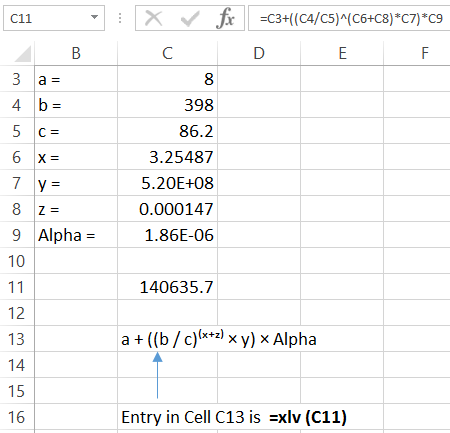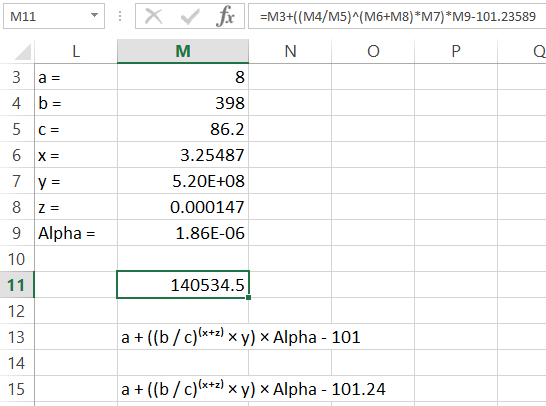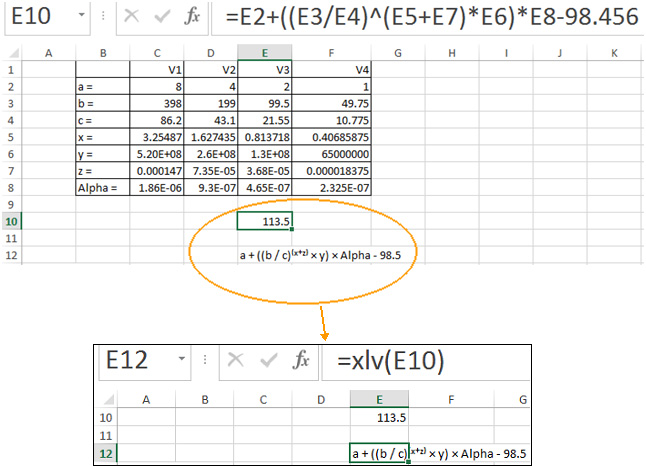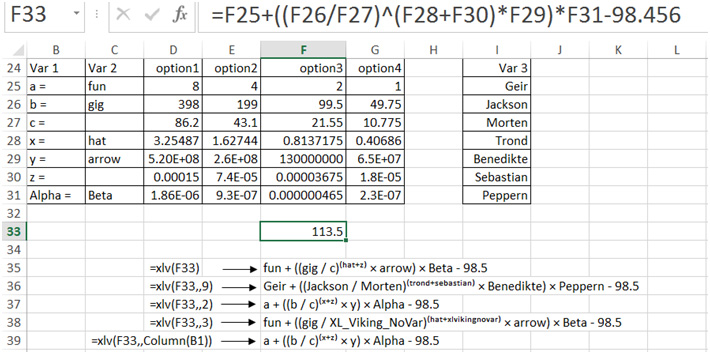# The XLV Function

## Function for displaying math formula with component variable names – xlv

=xlv(CELLREF, Optional Rounder(INTEGER), Optional ColumnNo(Integer))

This function displays the variable names of a mathematical formula defined in another cell. It updates automatically with a change in the values in the formula or a change in the formula.

Example

A formula (=C3+((C4/C5)^(C6+C8)*C7)*C9) is entered in Cell C11 that references the values in cells C3 to C9. Corresponding variable names are located in cells B3 to B9. Each variable name must be located in the same row as the value it is representing.

The variables of the formula in Cell C11 is shown in the cell where the xlv function is entered. In the example below, xlv is entered in Cell C13.Does the variable name need to be in the cell directly to the left of the value it is representing?
NO: By Default the xlv function looks for the first cell to the left of the value that is not a numeric value.

## How to use the Optional Parameters “Rounder” and “ColumnNo”

Rounder:
In the example above, the xlv function has been used without the Rounder parameter and so has defaulted to its adaptive formatting. The adaptive formatting works in the following way:

Each value in the formula is parsed in the following way:

if Value > 10000000 the number is expressed in Scientific notation
If 10000000 > Value > 100 the number in expressed in standard notation to zero decimal places
If 100 > Value > .001 the number in expressed in standard notation to 3 significant figures
if Value < .001 the number is expressed in Scientific notation

Generally, the xlv function will display the variables of the formula. However,  in an instance where a numeric value (rather than a CellRef) is entered in the formula  that the “xlv” function is referencing, the rounder parameter can be used to determine the number of decimal places to be displayed.

Example

The formula in cell M11 : =M3+((M4/M5)^(M6+M8)*M7)*M9-101.23589
The last term of the formula is 101.23589 and is a numeric value (and not a CellRef). By default, xlv uses the adaptive number formatting (as described above) and displays the result as shown below in Cell M13; Entry in Cell M13 is: =xlv(M11)

Cell M15 displays the result when setting the rounder parameter to 2 (i.e. 2 decimal places); Entry in Cell M15 is: =xlv(M11,2)ColumnNo:

By default, the xlv function returns the first cell to left of the value that is not a numeric value.

In the example below, a formula is entered in cell E10 that references the values in Column E.

=xlv(E10) is entered in cell E12. As can be seen by the output of the xlv function (in cell E12), the xlv function returns the variables located in Column B, since that is where the first non-numeric values are located.But what if I have more than one column with variable names to the left of the value I am referencing?

And;

What if my variable name is located to the right of the value I am referencing?

Use the second Optional Parameter “ColumnNo” to specify in which column the variable is located. See Below.

In the following example variables are located in Columns B, C and I. (A formula is located in Cell F33 and references values in Column F).

The xlv function is used in Cells F35 to F39 to demonstrate how the second optional parameter is used to specify in which column the variables are located.

The column number can be specified in various ways. E.G. “Column(B1)” can also be used to specify column number 2.Note 1: If there is no variable defined in the column that is specified, the xlv Function returns “XL_Viking_NoVar” or “xlvikingnovar” to indicate that no variable is defined.

Note 2: The second Optional variable is not used in the above example (hence the double commas), which means any numeric values that are entered in the formula will default to the XL-Viking adaptive number formatting, Ref “Rounder” Parameter.